/
/
/
61) 62) 63) 64) 65)
Not my Question
Flag Content

# Question : 61) 62) 63) 64) 65) : 1757186

61)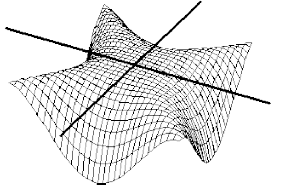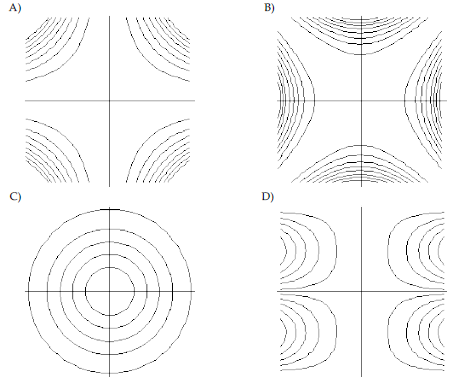62)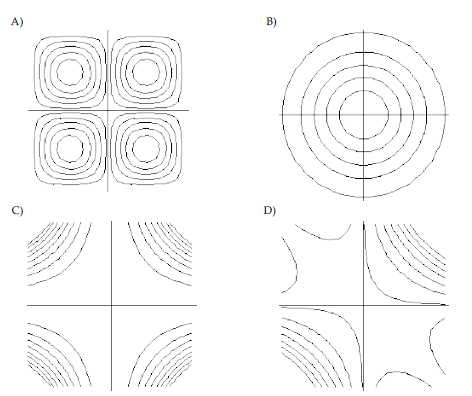63)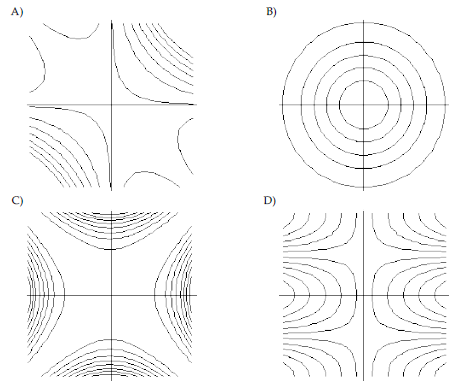64)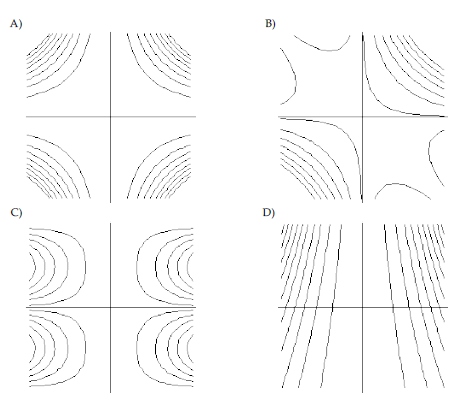65)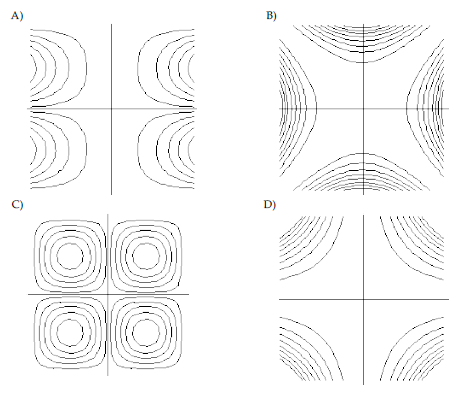66) According to the Gas Kinetic Theory, the average speed of a gas particle is given by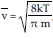, where v is the speed in m/s, k is the constant 1.38 × 10-23, T is the temperature of the gas in Kelvin, and m is the mass of the gas particle in kg. What is the average speed of an oxygen molecule with a mass of 5.314 × 10-26 kg at a temperature of 500 K?

A) 174 m/s B) 330,700 m/s C) 575 m/s D) 1020 m/s

67) The Ideal Gas Law states that the pressure P of a gas obeys the function P = f(V, n, T) = nkT/V, where n is the number of particles in the sample, T is the Kelvin temperature of the gas, V is the volume of the gas in liters, and k is the constant 1.38 × 10-23. Does the pressure have a local minimum along the line V = 4t, n = 3t, T = 2t? If so, what is the value of the minimum pressure? Give reasons for your answer.

68) A sample of matter has a temperature distribution given by T = f(x, y, z) = x2/5 + y2/5 + z2/4. Describe the level surfaces and write an equation for the surface T = 300.

## Solution 5 (1 Ratings )

Solved
Mathematics 3 Years Ago 72 Views